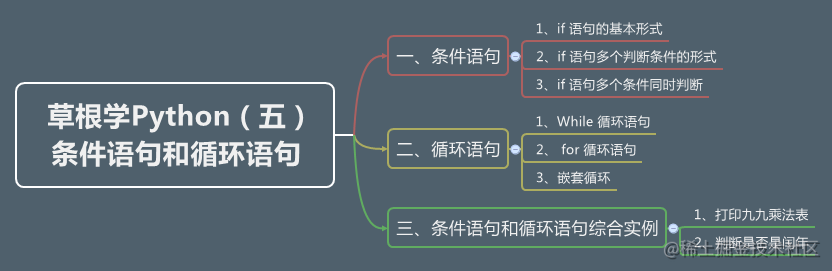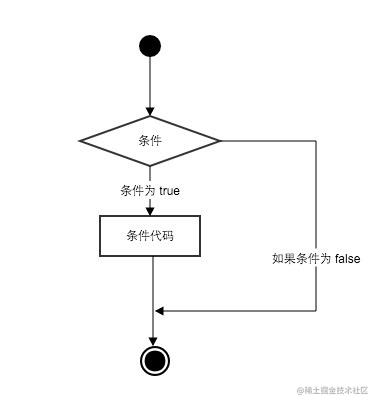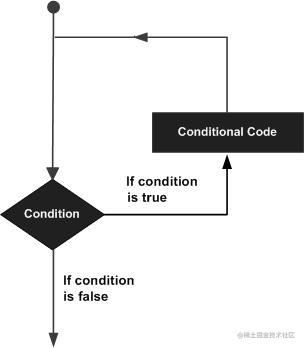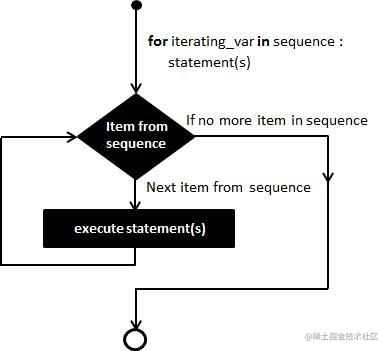# 目录# 一、条件语句

Python 条件语句跟其他语言基本一致的，都是通过一条或多条语句的执行结果（ True 或者 False ）来决定执行的代码块。

Python 程序语言指定任何非 0 和非空（null）值为 True，0 或者 null为 False。## 1、if 语句的基本形式

Python 中，if 语句的基本形式如下：

``````if 判断条件：
执行语句……
else：
执行语句……

if 语句的判断条件可以用>（大于）、<(小于)、==（等于）、>=（大于等于）、<=（小于等于）来表示其关系。

``````# -*-coding:utf-8-*-

results=59

if results>=60:
print ('及格')
else :
print ('不及格')

``````不及格

``````num = 6
if num :
print('Hello Python')

## 2、if 语句多个判断条件的形式

``````if 判断条件1:
执行语句1……
elif 判断条件2:
执行语句2……
elif 判断条件3:
执行语句3……
else:
执行语句4……

``````# -*-coding:utf-8-*-

results = 89

if results > 90:
print('优秀')
elif results > 80:
print('良好')
elif results > 60:
print ('及格')
else :
print ('不及格')

``````良好

## 3、if 语句多个条件同时判断

Python 不像 Java 有 switch 语句，所以多个条件判断，只能用 elif 来实现，但是有时候需要多个条件需同时判断时，可以使用 or （或），表示两个条件有一个成立时判断条件成功；使用 and （与）时，表示只有两个条件同时成立的情况下，判断条件才成功。

``````# -*-coding:utf-8-*-

java = 86
python = 68

if java > 80 and  python > 80:
print('优秀')
else :
print('不优秀')

if ( java >= 80  and java < 90 )  or ( python >= 80 and python < 90):
print('良好')

``````不优秀

# 二、循环语句Python 提供了 for 循环和 while 循环，当然还有一些控制循环的语句：

break在语句块执行过程中终止循环，并且跳出整个循环
continue在语句块执行过程中终止当前循环，跳出该次循环，执行下一次循环
passpass 是空语句，是为了保持程序结构的完整性

## 1、While 循环语句

``````count = 1
sum = 0
while (count <= 100):
sum = sum + count
count = count + 1
print(sum)

``````5050

``````count = 1
sum = 0
while (count <= 100):
sum = sum + count
if ( sum > 1000):  #当 sum 大于 1000 的时候退出循环
break
count = count + 1
print(sum)

``````1035

``````count = 1
sum = 0
while (count <= 100):
if ( count % 2 == 0):  # 双数时跳过输出
count = count + 1
continue
sum = sum + count
count = count + 1
print(sum)

``````2500

``````count = 0
while count < 5:
print (count)
count = count + 1
else:
print (count)

``````0
1
2
3
4
5

## 2、 for 循环语句

for循环可以遍历任何序列的项目，如一个列表或者一个字符串``````for iterating_var in sequence:
statements(s)

``````for letter in 'Hello 两点水':
print(letter)

``````H
e
l
l
o

``````for num in range(10,20):  # 迭代 10 到 20 之间的数字
for i in range(2,num): # 根据因子迭代
if num%i == 0:      # 确定第一个因子
j=num/i          # 计算第二个因子
print ('%d 是一个合数' % num)
break            # 跳出当前循环
else:                  # 循环的 else 部分
print ('%d 是一个质数' % num)

``````10 是一个合数
11 是一个质数
12 是一个合数
13 是一个质数
14 是一个合数
15 是一个合数
16 是一个合数
17 是一个质数
18 是一个合数
19 是一个质数

## 3、嵌套循环

Python 语言允许在一个循环体里面嵌入另一个循环。上面的实例也是使用了嵌套循环的，这里就不给出实例了。

for 循环嵌套语法

``````for iterating_var in sequence:
for iterating_var in sequence:
statements(s)
statements(s)

while 循环嵌套语法

``````while expression:
while expression:
statement(s)
statement(s)

# 三、条件语句和循环语句综合实例

## 1、打印九九乘法表

``````# -*- coding: UTF-8 -*-

# 打印九九乘法表
for i in range(1, 10):
for j in range(1, i+1):
# 打印语句中，大括号及其里面的字符 (称作格式化字段) 将会被 .format() 中的参数替换,注意有个点的
print('{}x{}={}\t'.format(i, j, i*j), end='')
print()

``````1x1=1
2x1=2   2x2=4
3x1=3   3x2=6   3x3=9
4x1=4   4x2=8   4x3=12  4x4=16
5x1=5   5x2=10  5x3=15  5x4=20  5x5=25
6x1=6   6x2=12  6x3=18  6x4=24  6x5=30  6x6=36
7x1=7   7x2=14  7x3=21  7x4=28  7x5=35  7x6=42  7x7=49
8x1=8   8x2=16  8x3=24  8x4=32  8x5=40  8x6=48  8x7=56  8x8=64
9x1=9   9x2=18  9x3=27  9x4=36  9x5=45  9x6=54  9x7=63  9x8=72  9x9=81

## 2、判断是否是闰年

``````
# 判断是否是闰年

year = int(input("请输入一个年份: "))
if (year % 4) == 0 and (year % 100) != 0 or (year % 400) == 0:
print('{0} 是闰年' .format(year))
else:
print('{0} 不是闰年' .format(year))